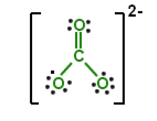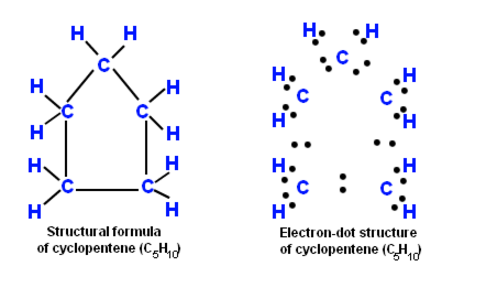Electron Dot Formula

Delocalized Bonding cannot be properly described by a single Electron Dot Formula. As an alternative, a resonance depiction is often used. Conferring to the resonance depiction, we can describe a molecules electron structure having delocalized bonding by noting down all conceivable Electron Dot Formula.

• A Lewis Electron Dot Formula comprises of one dot for every valence electron and an element’s symbol. Stages to articulate the electron dot formula are stated beneath.
• Note down a skeletal structure displaying a realistic bonding pattern by means of only the element symbols.
• Pick up every valence electrons from every atom and toss them into a make-believe container that we can term an electron pot.

Make use of the N – A = S equation to get to know the number of bonds

•       The sum of the number of valence electrons is equal to N
•       The number of valence electron in electron pot is A.
•       The number of electrons shared in the molecule equals S
•       Distribute the electrons from electron pot to account for the bonds
•       Distribute the rest of the electrons

Solved Examples

Problem 1: In terms of electron dot formulas, define the electron structure of the carbonate ion CO32-.

One potential electron dot formula for the carbonate ion isSince all carbon-oxygen bonds are likely to be equal, the electron structure in resonance terms is shown below.

Over the region of all three carbon-oxygen bonds, one electron pair is delocalized.

Question 2: Cyclopentane – What will be the formula and electron dot structure of this element?

Solution:

Single bonds connect five carbon atoms of cyclopentane in a cyclic form. The molecular formula of cyclopentane is C5H10. The electron dot structure and structural formula of cyclopentane are articulated underneath.Practise This Question

The spectrum from a black body radiation is a